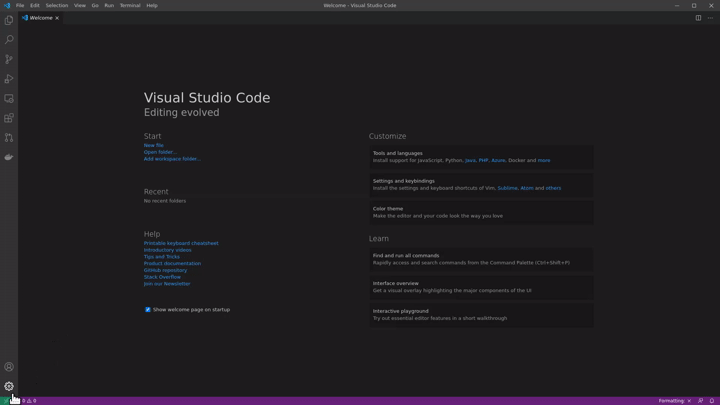# Gosl - Go scientific library

Gosl is a set of tools for developing scientific simulations using the Go language. We mainly consider the development of numerical methods and solvers for differential equations but also present some functions for fast Fourier transforms, the generation of random numbers, probability distributions, and computational geometry.

This library contains essential functions for linear algebra computations (operations between all combinations of vectors and matrices, eigenvalues and eigenvectors, linear solvers) and the development of numerical methods (e.g. numerical quadrature).

We link Gosl with existent libraries written in C and Fortran, such as OpenBLAS, LAPACK, UMFPACK, MUMPS, QUADPACK and FFTW3. These existing libraries have been fundamental for the development of high-performant simulations over many years. We believe that it is nearly impossible to rewrite these libraries in native Go and at the same time achieve the same speed delivered by them. Just for reference, a naive implementation of matrix-matrix multiplication in Go is more than 100 times slower than OpenBLAS.

## Installation

Because of the other libraries, the easiest way to work with Gosl is via Docker. Having Docker and VS Code installed, you can start developing powerful numerical simulations using Gosl in a matter of minutes. Furthermore, it works on Windows, Linux, and macOS out of the box.

### Containerized

1. Install Docker
2. Install Visual Studio Code
3. Install the Remote Development extension for VS Code
4. Clone https://awesomeopensource.com/project/cpmech/hello-gosl
5. Create your application within a container (see gif below)

Done. And your system will "remain clean."### Debian/Ubuntu GNU Linux

First, install Go as explained in https://golang.org/doc/install

Second, install some libraries:

``````sudo apt-get install \
gcc \
gfortran \
libfftw3-dev \
liblapacke-dev \
libmetis-dev \
libmumps-seq-dev \
libopenblas-dev \
libsuitesparse-dev
``````

``````git clone https://github.com/cpmech/gosl.git
cd gosl
./all.bash
``````

Done. Installation completed.

## Documentation

Gosl includes the following essential packages:

• chk. To check numerical results and for unit testing
• io. Input/output including printing to the terminal and handling files
• utl. To generate series (e.g. linspace) and other functions as in pylab/matlab/octave
• la. Linear Algebra: vector, matrix, efficient sparse solvers, eigenvalues, decompositions

Gosl includes the following main packages:

• fun. Special functions, DFT, FFT, Bessel, elliptical integrals, orthogonal polynomials, interpolators
• gm. Geometry algorithms and structures
• hb. Pseudo hierarchical binary (hb) data file format
• num. Fundamental numerical methods such as root solvers, non-linear solvers, numerical derivatives and quadrature
• ode. Solvers for ordinary differential equations
• pde. Solvers for partial differential equations (FDM, Spectral, FEM)
• rnd. Random numbers and probability distributions

## Previous version

The previous version, including more packages, is available here and can be used with the Docker image 1.1.3 as in this hello gosl example.

These other packages, such as machine learning, plotting, etc., have been removed because they do not depend on CGO and may be developed independently. We can now maintain the core of Gosl more efficiently, which has a focus on the foundation for other scientific code.

Get A Weekly Email With Trending Projects For These Topics
No Spam. Unsubscribe easily at any time.
Go (195,765
Optimization (2,478
Linear Algebra (819
Scientific Computing (619
Differential Equations (265
Computational Geometry (248
Linear Programming (232
Related Projects